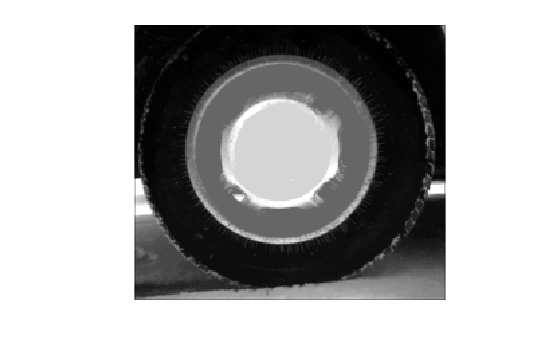# imfill

Fill image regions and holes

## Syntax

``BW2 = imfill(BW,locations)``
``BW2 = imfill(BW,locations,conn)``
``BW2 = imfill(BW,'holes')``
``BW2 = imfill(BW,conn,'holes')``
``I2 = imfill(I)``
``I2 = imfill(I,conn)``
``BW2 = imfill(BW)``
``BW2 = imfill(BW,0,conn)``
``[BW2, locations_out] = imfill(BW)``

## Description

example

````BW2 = imfill(BW,locations)` performs a flood-fill operation on background pixels of the input binary image `BW`, starting from the points specified in `locations`.```
````BW2 = imfill(BW,locations,conn)` fills the area defined by `locations`, where `conn` specifies the connectivity.```

example

````BW2 = imfill(BW,'holes')` fills holes in the input binary image `BW`. In this syntax, a hole is a set of background pixels that cannot be reached by filling in the background from the edge of the image.```

example

````BW2 = imfill(BW,conn,'holes')` fills holes in the binary image `BW`, where `conn` specifies the connectivity.```

example

````I2 = imfill(I)` fills holes in the grayscale image `I`. In this syntax, a hole is defined as an area of dark pixels surrounded by lighter pixels.```

example

````I2 = imfill(I,conn)` fills holes in the grayscale image `I`, where `conn` specifies the connectivity.```
````BW2 = imfill(BW)` displays the binary image `BW` on the screen and lets you define the region to fill by selecting points interactively with the mouse. To use this syntax, `BW` must be a 2-D image.Press Backspace or Delete to remove the previously selected point. Shift-click, right-click, or double-click to select a final point and start the fill operation. Press Return to finish the selection without adding a point.```
````BW2 = imfill(BW,0,conn)` lets you override the default connectivity as you interactively specify locations.```
````[BW2, locations_out] = imfill(BW)` returns the locations of points selected interactively in `locations_out`. To use this syntax, `BW` must be a 2-D image.```

## Examples

collapse all

```BW1 = logical([1 0 0 0 0 0 0 0 1 1 1 1 1 0 0 0 1 0 0 0 1 0 1 0 1 0 0 0 1 1 1 0 1 1 1 1 0 1 1 1 1 0 0 1 1 0 1 0 1 0 0 0 1 0 1 0 1 0 0 0 1 1 1 0]); BW2 = imfill(BW1,[3 3],8)```
```BW2 = 8x8 logical array 1 0 0 0 0 0 0 0 1 1 1 1 1 0 0 0 1 1 1 1 1 0 1 0 1 1 1 1 1 1 1 0 1 1 1 1 1 1 1 1 1 0 0 1 1 1 1 0 1 0 0 0 1 1 1 0 1 0 0 0 1 1 1 0 ```

```I = imread('coins.png'); figure imshow(I) title('Original Image')```Convert image to binary image.

```BW = imbinarize(I); figure imshow(BW) title('Original Image Converted to Binary Image')```Fill holes in the binary image and display the result.

```BW2 = imfill(BW,'holes'); figure imshow(BW2) title('Filled Image')``````I = imread('tire.tif'); I2 = imfill(I); figure, imshow(I), figure, imshow(I2)```## Input Arguments

collapse all

Binary image, specified as a logical array of any dimension.

Example: `BW = imread('text.png');`

Data Types: `logical`

Linear indices identifying pixel locations, specified as a numeric vector or 2-D numeric matrix of positive integers. If `locations` is a p-by-1 vector, then it contains the linear indices of the starting locations. If `locations` is a p-by-`ndims(BW)` matrix, then each row contains the array indices of one of the starting locations.

Example: `[3 3]`

Data Types: `double`

Grayscale image, specified as a numeric array of any dimension.

Example: ```I = imread('cameraman.tif');```

Data Types: `single` | `double` | `int8` | `int16` | `int32` | `int64` | `uint8` | `uint16` | `uint32` | `uint64` | `logical`

Pixel connectivity, specified as one of the values in this table. The default connectivity is `4` for 2-D images, and `6` for 3-D images.

Value

Meaning

Two-Dimensional Connectivities

4-connected

Pixels are connected if their edges touch. The neighborhood of a pixel are the adjacent pixels in the horizontal or vertical direction.8-connected

Pixels are connected if their edges or corners touch. The neighborhood of a pixel are the adjacent pixels in the horizontal, vertical, or diagonal direction.Three-Dimensional Connectivities

6-connected

Pixels are connected if their faces touch. The neighborhood of a pixel are the adjacent pixels in:

• One of these directions: in, out, left, right, up, and down18-connected

Pixels are connected if their faces or edges touch. The neighborhood of a pixel are the adjacent pixels in:

• One of these directions: in, out, left, right, up, and down

• A combination of two directions, such as right-down or in-up26-connected

Pixels are connected if their faces, edges, or corners touch. The neighborhood of a pixel are the adjacent pixels in:

• One of these directions: in, out, left, right, up, and down

• A combination of two directions, such as right-down or in-up

• A combination of three directions, such as in-right-up or in-left-downFor higher dimensions, `imfill` uses the default value `conndef(ndims(BW),'minimal')`.

Connectivity can also be defined in a more general way for any dimension by specifying a 3-by-3-by- ... -by-3 matrix of `0`s and `1`s. The `1`-valued elements define neighborhood locations relative to the center element of `conn`. Note that `conn` must be symmetric about its center element. See Specifying Custom Connectivities for more information.

Data Types: `double` | `logical`

## Output Arguments

collapse all

Filled binary image, returned as logical array.

Linear indices of pixel locations, returned as a numeric vector or matrix.

Filled grayscale image, returned as a numeric array.

## Algorithms

`imfill` uses an algorithm based on morphological reconstruction .

 Soille, P., Morphological Image Analysis: Principles and Applications, Springer-Verlag, 1999, pp. 173–174.